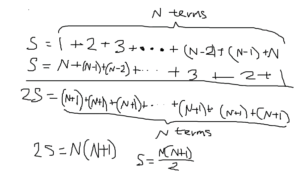(770) 852-0314 [email protected]
Select Page

# Why I Don’t Like Mathematical Induction

Everybody has opinions, and you can even have opinions about math. I, for one, don’t particularly like the method of mathematical induction. Why? Because it doesn’t completely tell you “why”. For example, you can use mathematical induction to prove that 1 + 2 + 3 + 4 + … + n = n(n+1)/2. Great, we know that the formula works, but where does the formula come from? A much more elegant method to prove that formula is derivation. There are many ways to derive that formula, and one method is by writing 1 + 2 + 3 + 4 + … + n forwards and backwards and by adding them together twice. That way, you can see how twice the sum would be n(n+1) (see the visual), so the sum would be n(n+1)/2. By deriving the formula, you see why the formula is the way it is, and you’ll be able to connect the formula to the nature of the series. Also, mathematical induction is not a method to make equations, as it can only be used if the equation is given. With induction, you may prove old equations, but with derivation, you’ll be able to make new equations.